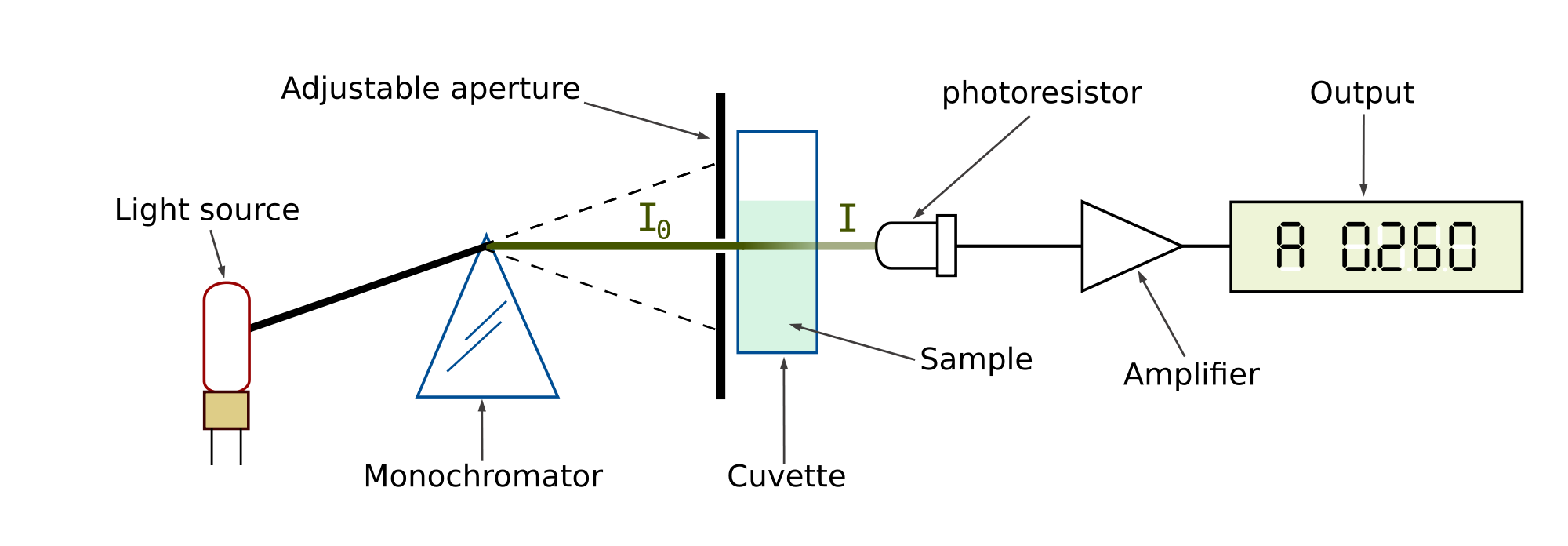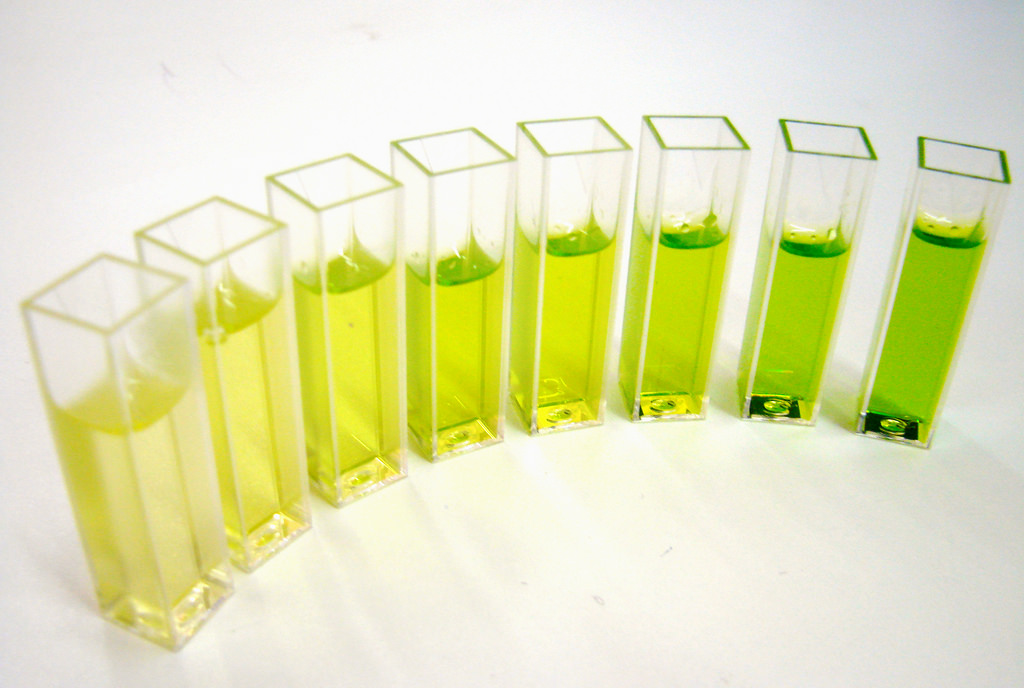# Spectrophotometer Working Principle

in #stemng3 years ago

The process of measuring the amount of light absorbed by a chemical substance when the intensity of light is been measured as the beam of light passes through the sample solution is referred to as spectrophotometry. Over a particular range of wavelength, compound do absorb or transmit light, this is the basic principle of spectrophotometry.

The measurement of this wavelength is very useful in measuring the amount of a known chemical substance. In quantitative analysis, spectrophotometry is always used. Spectrophotometry application is found in the field such as chemistry, biochemistry, physics, chemical and material engineering, and clinical applications.

The techniques of spectrophotometry can be used for any materials and chemical substances. For example, determination of enzyme catalyzed reactions in biochemistry makes use of the technique. This same technique in clinical application is used in the examination of tissues or blood for clinical diagnosis. Atomic absorption spectrophotometry and atomic emission spectrophotometry are the variations of spectrophotometer.

Spectrophotometer
An instrument that measures the amount of light (photons) in which a substance or sample absorbed after the light has passed through it is referred to as spectrophotometer. Determination of the amount of known chemical substance in concentration through the measurement of detected intensity of light is also one of the applications of spectrophotometry.Spectrophotometer skeleton

Spectrophotometry is classified into two based of the range of wavelength of the light source:

UV-visible spectrophotometer: It makes use of light over ultraviolet ray which is between 185-400nm and visible range which is between 400-700nm of electromagnetic radiation spectrum.

IR spectrophotometer: it makes use of light over infrared range which is between 700-1500nm of electromagnetic radiation spectrum.

In visible spectrophotometry, observed color is the means through which the determination of absorption or transmission of certain substance is done. For example, If sample solution transmit all visible wavelength i.e., it absorbs nothing, the sample solution appears white in theory, while if a solution sample absorbs light over all visible range, it appears black in theory.

Spectrophotometer is made up of the following structure, which includes; a light source, a monochromator, a collimator, a wavelength sector, a cuvette for solution sample, a photoelectric detector and a digital display (meter).

Working Principle of SpectrophotometerSpectrophotometer

A spectrophotometer is made up of two devices; a spectrometer and a photometer. Spectrometer produced light of different wavelength, while a photometer measures the intensity of the light produced. Note that the solution sample is placed between the spectrometer and the photometer.

Spectrometer: The device produced a desired wavelength range of light. The procedure is as follows; firstly, transmission of straight beam of light by collimator which passes through a monochromator. The monochromator which is also referred to as prism is used to split the light beam into different wavelength and the wavelength selector transmit only the wavelength that are desired.

Photometer: Since the solution sample is placed between the spectrometer and photometer, after a light beam of different wavelength is produced in spectrometer, the light beam passes through the solution sample in the cuvette and photometer measures the amount of photon absorbed and delivers the signal (result) to the digital display.cuvette

When the intensity of light after it is transmitted through the cuvette is known, it can be related to the transmittance (T).The fraction of light that is transmitted through a solution sample is referred to as Transmittance (T).

Equation for calculating Transmittance(T);
Transmittance T =l/l0
Where l = light intensity after light beam is transmitted through the sample solution in the cuvette
And l0 = light intensity before the light is transmitted through the solution in the cuvette
Transmittance and absorbance are related with this expression;
Absorbance (A) = −log(T) = −log(l/l0)

Amount of photons absorbed is referred to as absorbance. Concentration of a sample can be calculated with known amount of absorbance by using Beer-Lambert law.

Beer- Lambert law

Beer-Lambert law is also known as Beer’s law. The law states that there is a linear relationship between the absorbance and concentration of a sample. The Beer’s law is effective when a linear relationship exist between absorbance and concentration.
Beer’s law is written as A= ϵlc

Where
A= Amount of absorbance
ϵ = Absorption coefficient (molar extinction coefficient)
l =Path length
c = concentration
Absorption coefficient is different from one molecule to the other and it’s given as a constant. The unit of ϵ is Lmol-1cm-1. The length path is measured in centimeter, the absorption has no unit and concentration is measured in mol/dm3

References
1
2
3
4
5

STEEM 1.31
TRX 0.12
JST 0.139
BTC 59563.20
ETH 2140.11
BNB 486.38
SBD 8.73